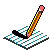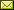PHYSICS@HORTONPhysics12

Physics 11

Math 11

NS Exams

Curriculum

Tips

Tutorials

McGraw Hill

Resources

Need it?

News

Enrichment

GiancoliIB Physics SL

November 2015 Notes

 November 2 Forces (Resultant Forces/Balanced & Unbalanced Forces/Newton's First Law/Translational Equilibrium/Newton's Second Law) Notes Forces (Newton's Second Law) BrainPop: Force Forces & Motion: Basics (Tug of War & Acceleration Lab) (needs Java) Notes November 3 Forces (Friction) The Physics Classroom The Calculator Pad Forces (Fluid Resistance) Notes November 4 Forces in 1D (needs Java) Work on "TO DO" list November 5 Momentum & Impulse (Momentum, Impulse & Newton's Second Law/F-t graphs/Conservation of Linear Momentum) Momentum & Impulse More Momentum & Impulse Impulse Bouncing Ball Momentum & Collisions (PHYSCLIPS) Notes November 6 Momentum & Impulse (Conservation of Linear Momentum) Finish Popper Physics pHET Collision Lab (needs JAVA!!! ~ settings: 1D, check velocity vectors & momenta diagram, try 100%, 50%, 0% elasticity, try different mass combinations, try different velocities and look at the total mometum before & after collision) Notes Momentum & Impulse (Conservation of Linear Momentum) Cannon Ball Fired from Back of Moving Truck Notes November 9 Momentum & Impulse (Conservation of Linear Momentum) Notes Video: Momentum (Vector Addition) 2D Collisions (World-in-Motion video analysis) Another Example November 10 Remembrance Day Assembly November 12 Momentum & Impulse (Newton's Third Law) Notes November 13 QUIZ (forces & impulse/momentum) Work, power, and energy (Forms of energy, conservation of energy, work) BrainPop: Work BrainPop: Inclined Plane Notes November 16 Work, power, and energy (Work, force displacement graphs, Hooke's Law) Absorb Physics: Doing Work Hooke's Law Animation Hooke's Law Spring Virtual Hooke's Law Lab Hooke's Law (showing elastic limit) Notes November 17 Work, power, and energy (Kinetic energy, gravitational potential energy, energy transformations) BrainPop: Kinetic Energy BrainPop: Potential Energy Energy of a Tossed Ball Energy Conservation in a Pendulum Energy Conservation in a Roller Coaster Notes November 18 Work, power, and energy (Elastic Collisions) Notes November 19 Work, power, and energy (Power & efficiency) BrainPop: Power Notes November 20November 20 Work Day/Review of TOPIC 2 November 23 Thermal Concepts (temperature & the Kelvin scale) Boyle's Law & Boyle's Law animation Charles's Law Gay Lussac's Law (Pressure Law) Gas Laws Kelvin Temperature Scale Absolute Zero: A Sense of Scale Bose-Einstein Condensate Notes November 24 Go over quiz Thermal Concepts (internal energy & macroscopic/microscopic concepts) Internal Energy (Hyper Physics) Components of Internal Energy Thermal Concepts (the mole, molar mass & Avogadro's constant) The Mole & Molar Mass Demystifying the Mole (see picture of moles of different sunstances) Notes November 25 Work day November 30 QUIZ (work, power & energy) Review of TOPIC 2

{subdirectory}